# b) A causal LTI system is described by the following difference equation: y(n) – Ay(n-1) -...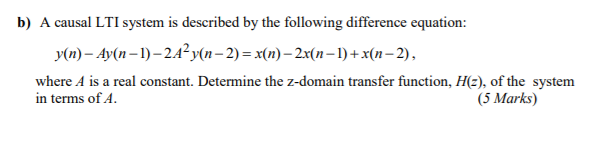b) A causal LTI system is described by the following difference equation: y(n) – Ay(n-1) - 24y(n − 2) = x(n) – 2x(n-1) + x(n–2), where A is a real constant. Determine the z-domain transfer function, Hz), of the system in terms of A. (5 Marks)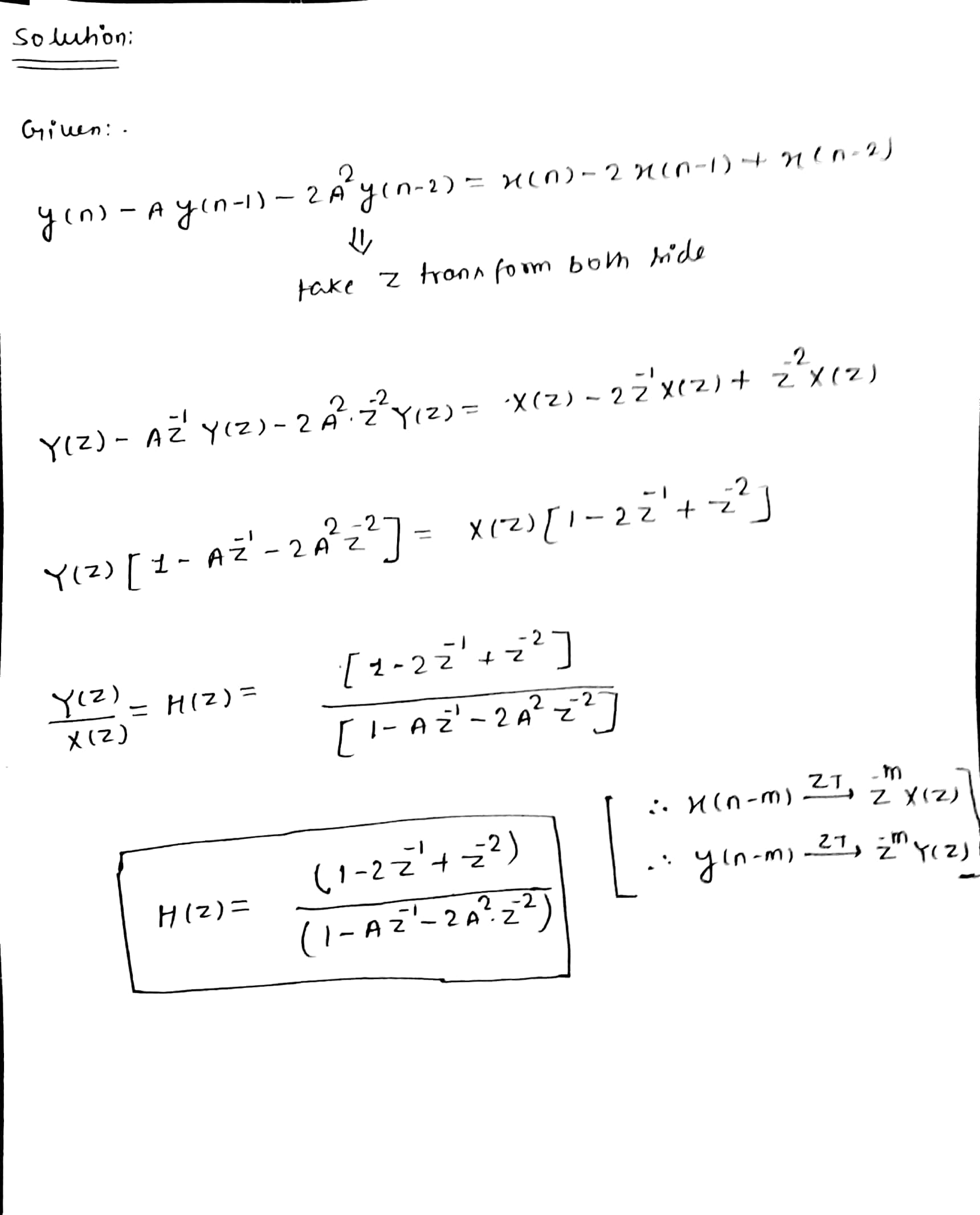#### Earn Coin

Coins can be redeemed for fabulous gifts.

Similar Homework Help Questions
• ### 5. (20 points) For a causal LTI discrete-time system described by the difference equation: y[n] +...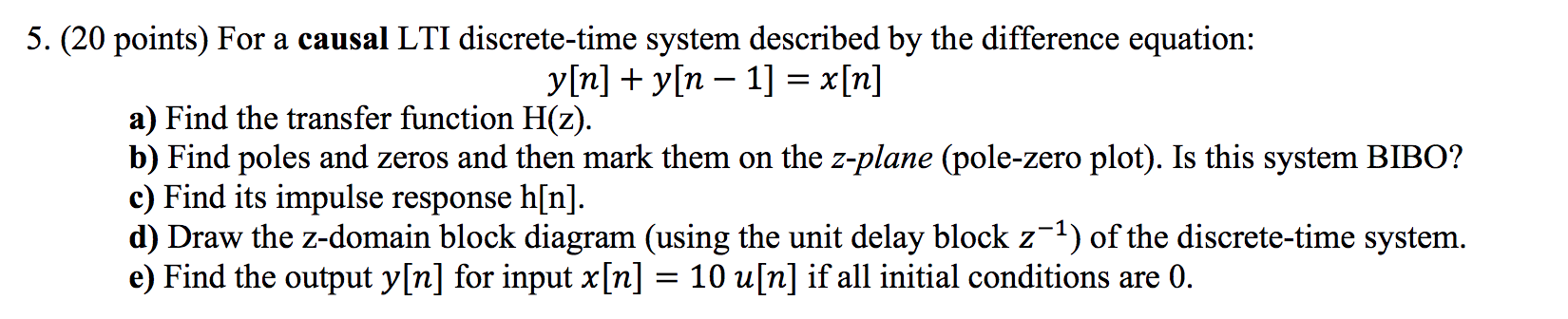5. (20 points) For a causal LTI discrete-time system described by the difference equation: y[n] + y[n – 1] = x[n] a) Find the transfer function H(z). b) Find poles and zeros and then mark them on the z-plane (pole-zero plot). Is this system BIBO? c) Find its impulse response h[n]. d) Draw the z-domain block diagram (using the unit delay block z-1) of the discrete-time system. e) Find the output y[n] for input x[n] = 10 u[n] if all...

• ### 1) A causal discrete-time system is described by the difference equation, y(n) = x(n)+3x(n-1)+ 2x(n-4) a)...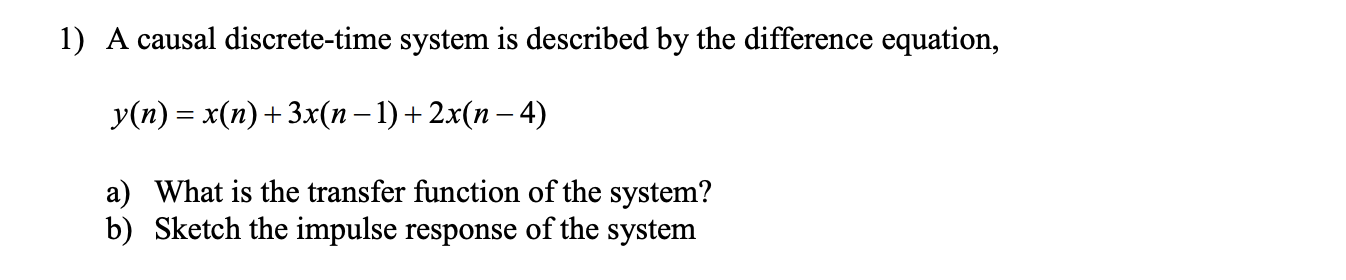1) A causal discrete-time system is described by the difference equation, y(n) = x(n)+3x(n-1)+ 2x(n-4) a) What is the transfer function of the system? b) Sketch the impulse response of the system

• ### Consider a discrete-time system described by the following diﬀerence equation. y(n) = y(n−1)−.24y(n−2) + 2x(n−1)−1.6x(n−2) Find...

Consider a discrete-time system described by the following diﬀerence equation. y(n) = y(n−1)−.24y(n−2) + 2x(n−1)−1.6x(n−2) Find the transfer function H(z). Find the zero-state response to the causal exponential input x(k) = .8nµ(n). This means that given H(z), we can calculate Y(z) and subsequently the output, y(n) with all initial conditions presumed to be zero. Hence the term, zero-state.

• ### Consider an LTI system whose input x[n] and output y[n] are related by the difference equation...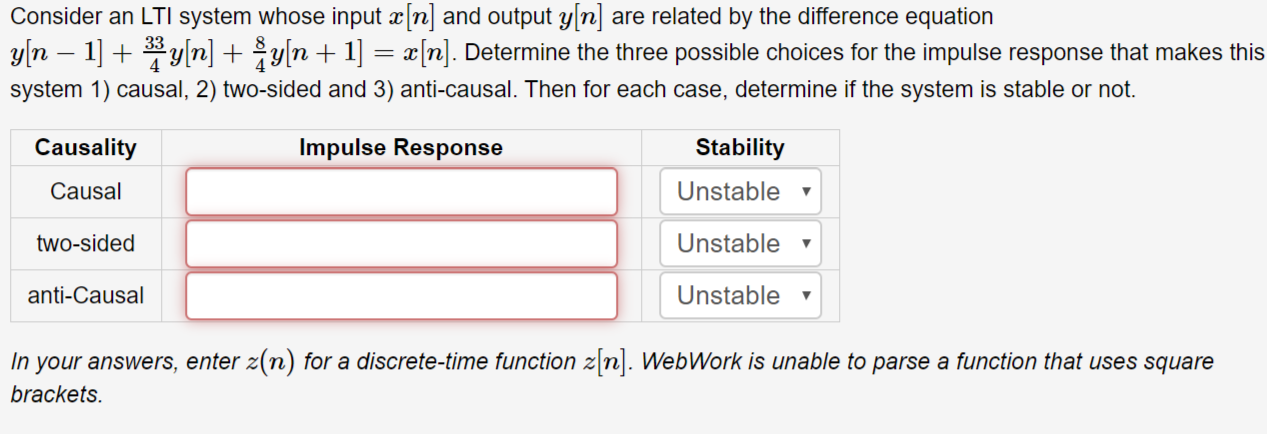Consider an LTI system whose input x[n] and output y[n] are related by the difference equation y[n – 1] + 3 y[n] + \$y[n + 1] = x[n]. Determine the three possible choices for the impulse response that makes this system 1) causal, 2) two-sided and 3) anti-causal. Then for each case, determine if the system is stable or not. Causality Impulse Response Stability Causal Unstable v two-sided Unstable anti-Causal Unstable y In your answers, enter z(n) for a discrete-time...

• ### 2. A discrete time LTI system is described by the difference equation (assume initial conditions are...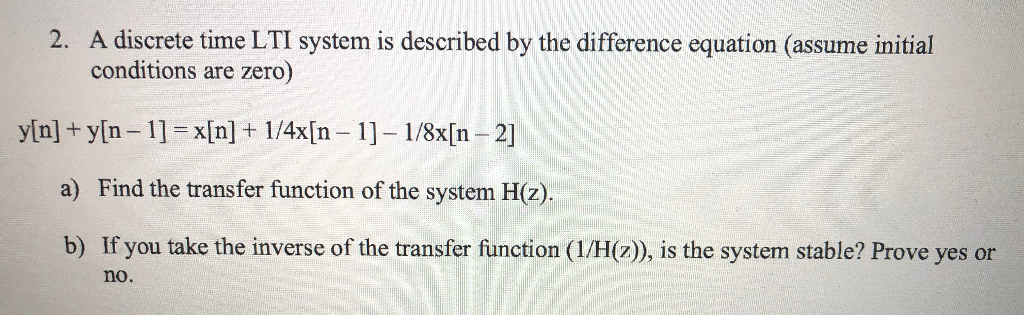2. A discrete time LTI system is described by the difference equation (assume initial conditions are zero) y[n] + y[n – 1] = x[n] + 1/4x[n – 1] – 1/8x[n – 2] a) Find the transfer function of the system H(z). b) If you take the inverse of the transfer function (1/H(z)), is the system stable? Prove yes or no.

• ### 1.9.10 A causal discrete-time LTI system is described by the equation 1 1 1 vn)(n) I(n-...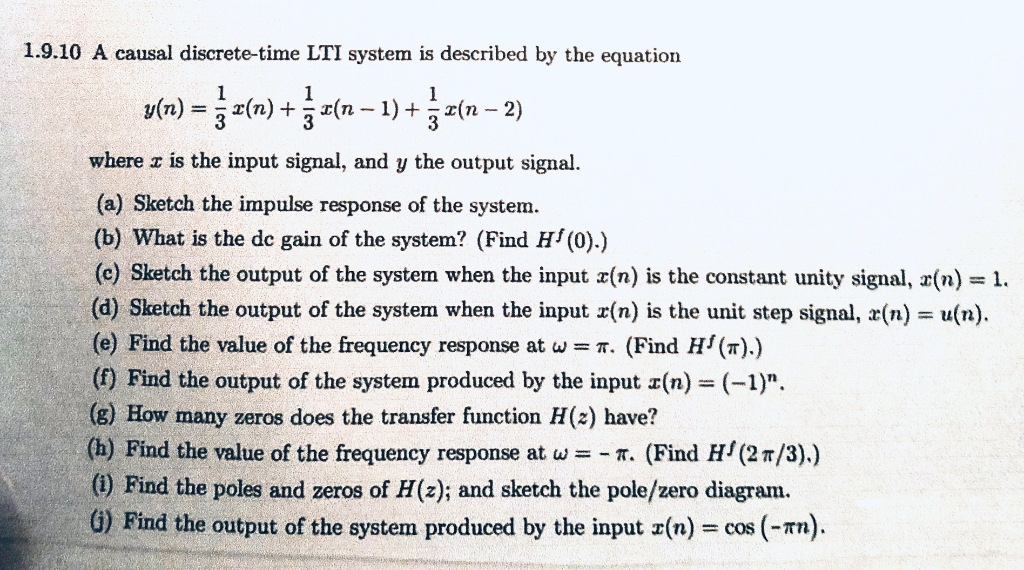1.9.10 A causal discrete-time LTI system is described by the equation 1 1 1 vn)(n) I(n- 1)+ 3 2(n 2) 3 3 where z is the input signal, and y the output signal (a) Sketch the impulse response of the system. (b) What is the de gain of the system? (Find H (0).) (c) Sketch the output of the system when the input x(n) is the constant unity signal, r(n) = 1. (d) Sketch the output of the system when...

• ### Problem 4. (20 points): Consider a causal LTI system that is described by the difference equation...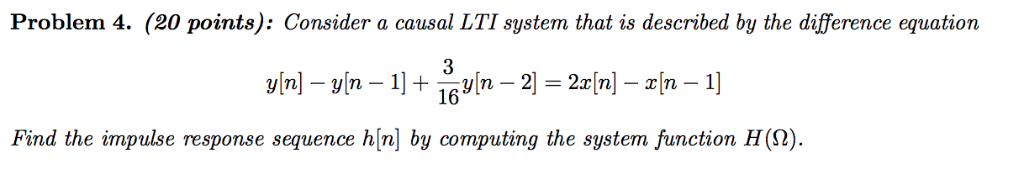Problem 4. (20 points): Consider a causal LTI system that is described by the difference equation Find the impulse response sequence h[n] by computing the system function H(S2)

• ### 7. A causal LTI system has a transfer function given by H (z) = -1 (1...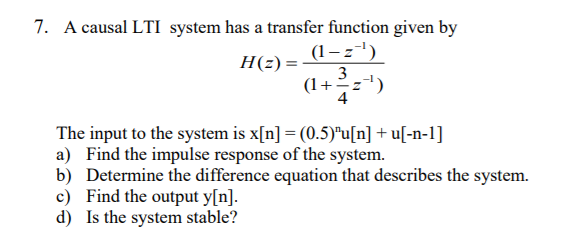7. A causal LTI system has a transfer function given by H (z) = -1 (1 4 The input to the system is x[n] = (0.5)"u[n] + u[-n-1] ) Find the impulse response of the system b) Determine the difference equation that describes the system. c) Find the output y[n]. d) Is the system stable?

• ### a causal discrete time LTI system is implemented using the difference equation y(n)-0.5y(n-1)=x(n)+x(n-1) where x(n) is...

a causal discrete time LTI system is implemented using the difference equation y(n)-0.5y(n-1)=x(n)+x(n-1) where x(n) is the input signal and y(n) the output signal. Find and sketch the impulse response of the system

• ### (2) Consider the causal discrete-time LTI system with an input r (n) and an output y(n)...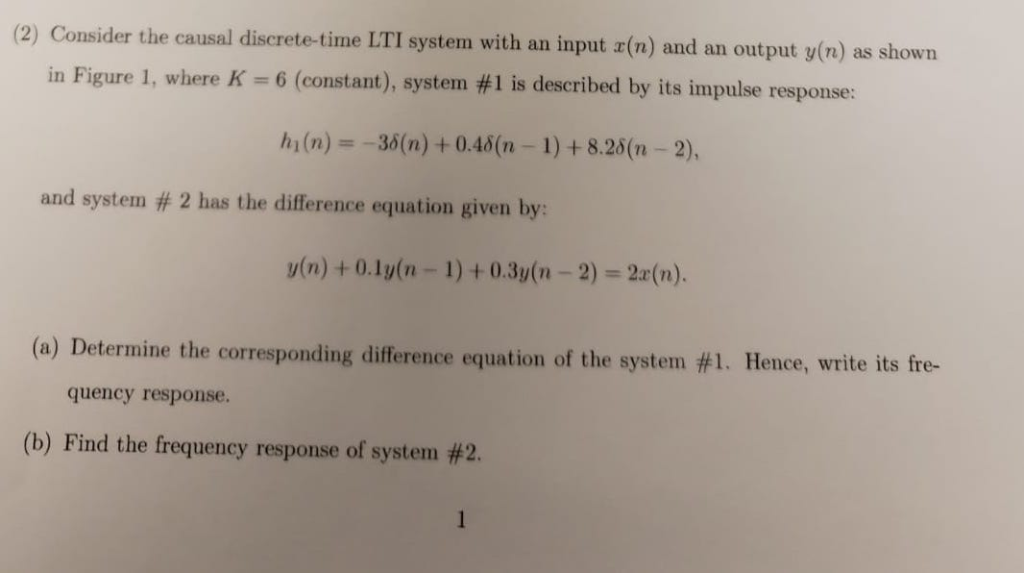(2) Consider the causal discrete-time LTI system with an input r (n) and an output y(n) as shown in Figure 1, where K 6 (constant), system #1 is described by its impulse response: h(n) = -36(n) + 0.48(n- 1)+8.26(n-2), and system # 2 has the difference equation given by: y(n)+0.1y(n-1)+0.3y(n-2)- 2a(n). (a) Determine the corresponding difference equation of the system #1. Hence, write its fre- quency response. (b) Find the frequency response of system #2. 1 system #1 system #2...